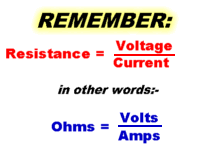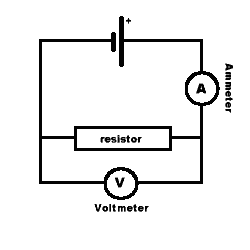## Electrical Resistance1 If the Voltmeter reading is 12V, and the Ammeter reading is 3A, what is the resistance? 36 Ohms 12 Ohms 4 Ohms 3 Ohms 4 Volts 2 What is the value of the resistor if a current of 2A flows when 8V is applied? 16 Ohms 8 Ohms 4 Ohms 2 Ohms 3 Now you need to turn the equation around. How much current will flow through a 10 Ohm resistor when 30V is applied? 3 Amps 10 Volts 10 Ohms 30 Amps 300 Amps 4 What voltage is required to cause a current of 0.5A to flow through a 10 Ohm resistor? 0.5 Volts 5 Volts 20V 20 Ohms 5 Ohms